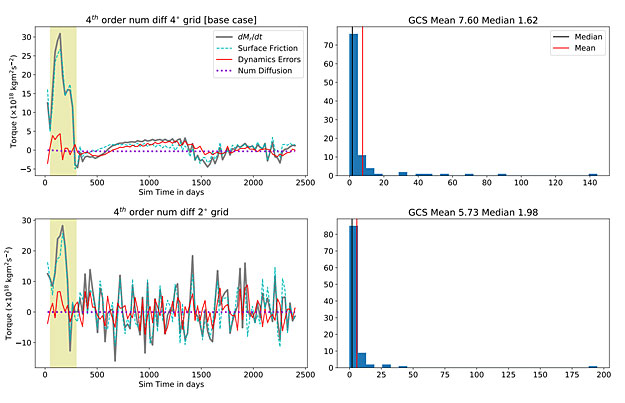# General Circulation Model Errors Are Variable Across Exoclimate Parameter Spaces

astro-ph.EP
October 31, 2021
Filed underThe first two rows of 3 are shown here with the histogram of the quantity F/(D + ) calculated at each output timestep which is used to calculate the GCS through Eqn. 8. The right panels show the mean and median of the distribution of these ratios, which were used to calculate two possible GCS values.

General circulation models are often used to explore exoclimate parameter spaces and classify atmospheric circulation regimes.

Models are tuned to give reasonable climate states for standard test cases, such as the Held-Suarez test, and then used to simulate diverse exoclimates by varying input parameters such as rotation rates, instellation, atmospheric optical properties, frictional timescales and so on. In such studies, there is an implicit assumption that the model which works reasonably well for the standard test case will be credible at all points in an arbitrarily wide parameter space.

Here, we test this assumption using the open-source general circulation model THOR to simulate atmospheric circulation on tidally locked Earth-like planets with rotation periods of 0.1 to 100 days. We find that the model error, as quantified by the ratio between physical and spurious numerical contributions to the angular momentum balance, is extremely variable across this range of rotation periods with some cases where numerical errors are the dominant component. Increasing model grid resolution does improve errors but using a higher-order numerical diffusion scheme can sometimes magnify errors for finite-volume dynamical solvers.

We further show that to minimize error and make the angular momentum balance more physical within our model, the surface friction timescale must be smaller than the rotational timescale.

Pushkar Kopparla, Russell Deitrick, Kevin Heng, João M. Mendonça, Mark Hammond

Comments: 8 pages, 5 figures, accepted by ApJ
Subjects: Earth and Planetary Astrophysics (astro-ph.EP); Atmospheric and Oceanic Physics (physics.ao-ph)
Cite as: arXiv:2110.10925 [astro-ph.EP] (or arXiv:2110.10925v1 [astro-ph.EP] for this version)
Submission history
From: Pushkar Kopparla
[v1] Thu, 21 Oct 2021 06:45:38 UTC (227 KB)
https://arxiv.org/abs/2110.10925
Astrobiology,SpaceRef co-founder, Explorers Club Fellow, ex-NASA, Away Teams, Journalist, Space & Astrobiology, Lapsed climber.